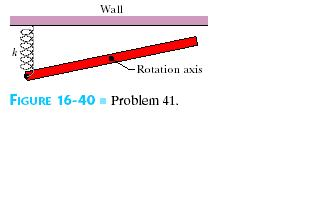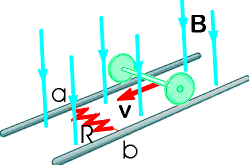Ask question

# In the overhead view of, a long uniform rod of mass m0.6 Kg is free to rotate in a horizontal planeabout a vertical axis through its center .A spring with force constant k = 1850 N/m is connected horizontally betweenone end of the rod and a fixed wall. When the rod is in equilibrium, it is parallel to the wall. What isthe period of the small oscillations thatresult when the rod is rotated slightly and released?# In the overhead view of, a long uniform rod of mass m0.6 Kg is free to rotate in a horizontal planeabout a vertical axis through its center .A spring with force constant k = 1850 N/m is connected horizontally betweenone end of the rod and a fixed wall. When the rod is in equilibrium, it is parallel to the wall. What isthe period of the small oscillations thatresult when the rod is rotated slightly and released?

Question
Otherasked 2021-05-10
In the overhead view of, a long uniform rod of mass m0.6 Kg is free to rotate in a horizontal planeabout a vertical axis through its center .A spring with force constant k = 1850 N/m is connected horizontally betweenone end of the rod and a fixed wall. When the rod is in equilibrium, it is parallel to the wall. What isthe period of the small oscillations thatresult when the rod is rotated slightly and released?## Answers (1)2021-05-12
In theoverhead view of , a long uniform rodof mass ( m = 0.6 Kg) is freeto rotate in a horizontal plane about avertical axis through its center.
A spring with force constant( k = 1850 N/m ) is connected horizontally between one end of the rod and a fixed wall.
When the rod is inequilibrium, it is parallel to the wall.
Let ' T ' be the time-period of the small oscillations that result when the rod is rotated slightly and released
Let rod ( length L) rotates by angle (PKS\thetaZSK)
Let 'F ' be the force applied on the rod, then spring elongated by $$\displaystyle{\left(\triangle{x}\right)}={\frac{{{L}\theta}}{{{2}}}}$$
Since , the moment of force $$\displaystyle{\left(\tau\right)}=-{\frac{{{F}{L}}}{{{2}}}}$$
Or $$\displaystyle{\left(\tau=-{\frac{{{F}{L}}}{{{2}}}}=-{\frac{{{k}{L}\theta}}{{{2}}}}\cdot{\frac{{{L}}}{{{2}}}}\right.}$$
$$\displaystyle=-{\frac{{{k}{L}^{{2}}}}{{{4}}}}\cdot\theta$$
So, the time period $$\displaystyle{\left({T}\right)}={2}\pi\cdot\sqrt{{{\frac{{{l}}}{{{C}}}}}}$$
where $$\displaystyle{I}={\frac{{{m}{L}^{{2}}}}{{{12}}}}$$
$$\displaystyle{C}={\frac{{{k}{L}^{{2}}}}{{{4}}}}$$
So, $$\displaystyle{T}={2}\pi\cdot\sqrt{{{\frac{{{m}}}{{{3}{k}}}}}}$$
Here m=0.6 kg and k=1850 N/m
T=0.06533s

### Relevant Questionsasked 2021-03-24
A 2.4-kg object is attached to a horizontal spring of forceconstant k=4.5 kN/m. The spring is stretched 10 cm fromequilibrium and released. Find (a) the frequency of themotion, (b) the period, (c) the amplitude, (d) the maximum speed,and (e) the maximum acceleration. (f) When does the objectfirst reach its equilibrium position? What is itsacceleration at this time?
Two identical blocks placed one on top of the other rest on africtionless horizontal air track. The lower block isattached to a spring of spring constant k= 600 N/m. Whendisplaced slightly from its equilibrium position, the systemoscillates with a frequency of 1.8 Hz. When the amplitude ofoscillation exceeds 5 cm, the upper block starts to slide relativeto the lower one. (a) What are the masses of the twoblocks? (b) What is the coefficient of static frictionbetween the two blocks?asked 2021-02-19
A 10 kg objectexperiences a horizontal force which causes it to accelerate at 5 $$\displaystyle\frac{{m}}{{s}^{{2}}}$$, moving it a distance of 20 m, horizontally.How much work is done by the force?
A ball is connected to a rope and swung around in uniform circular motion.The tension in the rope is measured at 10 N and the radius of thecircle is 1 m. How much work is done in one revolution around the circle?
A 10 kg weight issuspended in the air by a strong cable. How much work is done, perunit time, in suspending the weight?
A 5 kg block is moved up a 30 degree incline by a force of 50 N, parallel to the incline. The coefficient of kinetic friction between the block and the incline is .25. How much work is done by the 50 N force in moving the block a distance of 10 meters? What is the total workdone on the block over the same distance?
What is the kinetic energy of a 2 kg ball that travels a distance of 50 metersin 5 seconds?
A ball is thrown vertically with a velocity of 25 m/s. How high does it go? What is its velocity when it reaches a height of 25 m?
A ball with enough speed can complete a vertical loop. With what speed must the ballenter the loop to complete a 2 m loop? (Keep in mind that the velocity of the ball is not constant throughout the loop).asked 2021-02-23
A 0.30 kg ladle sliding on a horizontal frictionless surface isattached to one end of a horizontal spring (k = 500 N/m) whoseother end is fixed. The ladle has a kinetic energy of 10 J as itpasses through its equilibrium position (the point at which thespring force is zero).
(a) At what rate is the spring doing work on the ladle as the ladlepasses through its equilibrium position?
(b) At what rate is the spring doing work on the ladle when thespring is compressed 0.10 m and the ladle is moving away from theequilibrium position?asked 2021-05-04
Assume that a 1.00-kg ball is thrown solely by the action of the forearm, which rotates about the elbow joint under the action of the triceps muscle. The ball is accelerated uniformly from rest to 10.0 m/s in 0.350 s, at which point it is released. Calculate (a) the angular acceleration of the arm, and (b) the force required of the triceps muscle. Assume that the forearm has a mass of 3.70 kg and rotates like a uniform rod about an axis at its end.asked 2021-02-19
An airplane propeller is 2.08 m in length (from tip to tip) and has a mass of 117 kg. When the airpline's engine is first started, it applies a constant torque of $$\displaystyle{1950}\ {N}\cdot{m}$$ to the propeller, which starts from rest.
a) What is the angular acceleration of the propeller? Model the propeller as a slender rod.
b) What is the propeller's angular speed after making 5.00 revolutions?
c) How much work is done by the engine during the first 5.00 revolutions?
e) What is the instantaneous power output of the motor at the instant that the propeller has turne through 5.00 revolutions?asked 2021-03-21
In the figure below, the rolling axle, 1.43 m long, is pushed along horizontal rails at a constant speed v = 3.36 m/s.A resistor R = 0.325 ohm is connected to the rails at points a and b, directly opposite each other. (The wheels make good electrical contact with the rails, and so the axle, rails, and R form a closed-loop circuit. The only significant resistance in the circuit is R.) There is a uniform magnetic field B = 0.0850 T vertically downward. Calculate the induced current I in the resistor and what horizontal force F is required to keep the axle rolling at constant speed?asked 2021-04-13
As depicted in the applet, Albertine finds herself in a very odd contraption. She sits in a reclining chair, in front of a large, compressed spring. The spring is compressed 5.00 m from its equilibrium position, and a glass sits 19.8m from her outstretched foot.
a)Assuming that Albertine's mass is 60.0kg , what is $$\displaystyle\mu_{{k}}$$, the coefficient of kinetic friction between the chair and the waxed floor? Use $$\displaystyle{g}={9.80}\frac{{m}}{{s}^{{2}}}$$ for the magnitude of the acceleration due to gravity. Assume that the value of k found in Part A has three significant figures. Note that if you did not assume that k has three significant figures, it would be impossible to get three significant figures for $$\displaystyle\mu_{{k}}$$, since the length scale along the bottom of the applet does not allow you to measure distances to that accuracy with different values of k.asked 2020-11-14
A spring of negligible mass stretches 3.00 cm from its relaxed length when a force of 8.50 N is applied. A 0.530-kg particle rests on a frictionless horizontal surface and is attached to the free end of the spring. The particle is displaced from the origin to x = 5.00 cm and released from rest at t = 0. (Assume that the direction of the initial displacement is positive.)
(a) What is the force constant of the spring? 280 N/m
(b) What are the angular frequency (?), the frequency, and the period of the motion?
? = 23.121 rad/s
f = 3.6817 Hz
T = 0.27161 s
(c) What is the total energy of the system? 0.35 J
(d) What is the amplitude of the motion? 5 cm
(e) What are the maximum velocity and the maximum acceleration of the particle?
$$\displaystyle{v}_{{\max}}={1.1561}\frac{{m}}{{s}}$$
$$\displaystyle{a}_{{\max}}={26.73}\frac{{m}}{{s}^{{{2}}}}$$
(f) Determine the displacement x of the particle from the equilibrium position at t = 0.500 s.
(g) Determine the velocity and acceleration of the particle when t = 0.500 s. (Indicate the direction with the sign of your answer.)
v = _________________ $$\displaystyle\frac{{m}}{{s}}$$
a = _________________ $$\displaystyle\frac{{m}}{{s}^{{{2}}}}$$asked 2021-05-12
4.7 A multiprocessor with eight processors has 20attached tape drives. There is a large number of jobs submitted tothe system that each require a maximum of four tape drives tocomplete execution. Assume that each job starts running with onlythree tape drives for a long period before requiring the fourthtape drive for a short period toward the end of its operation. Alsoassume an endless supply of such jobs.
a) Assume the scheduler in the OS will not start a job unlessthere are four tape drives available. When a job is started, fourdrives are assigned immediately and are not released until the jobfinishes. What is the maximum number of jobs that can be inprogress at once? What is the maximum and minimum number of tapedrives that may be left idle as a result of this policy?
b) Suggest an alternative policy to improve tape driveutilization and at the same time avoid system deadlock. What is themaximum number of jobs that can be in progress at once? What arethe bounds on the number of idling tape drives?asked 2021-03-31
A paraglider is flying horizontally at a constant speed.Assume that only two forces act on it in the vertical direction,its weight and a vertical lift force exerted on its wings by theair. The lift force has a magnitude of 1800 N.
(a) What is the magnitude and direction of the force that theparaglider exerts on the earth ?
(b)If the lift force should suddenly decrease to 1200 N, whatwould be the vertical acceleration of the glider ? For bothquestions, take the upward direction to be the + y direction.
...